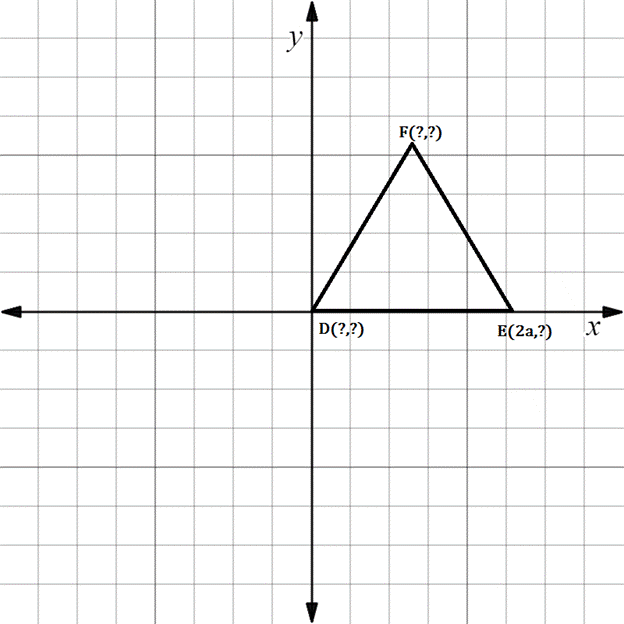Chapter 10.CT, Problem 13CTElementary Geometry For College St...

7th Edition
Alexander + 2 others
ISBN: 9781337614085

Solutions

Chapter
SectionElementary Geometry For College St...

7th Edition
Alexander + 2 others
ISBN: 9781337614085
Textbook Problem

Using as few variables as possible, state the coordinates of each point if △ D E F is isosceles with ≅ .D E F is an isosceles triangle with ≅

To determine

To find:

The missing coordinates for the vertices.

Explanation

The given figure is shown below.

From the figure, the following observations are made.

Since, the vertex D is at the origin.

The coordinates of D0, 0.

Now, consider the vertex E.

Here, x-coordinate is given as 2a.

In the E vertex, observe where it lies on y- axis.

Since, it is at 0, the vertex E has the coordinates 2a, 0.

The distance from D to E in the x- axis is 2a.

F lies midway between D and E

Still sussing out bartleby?

Check out a sample textbook solution.

See a sample solution

The Solution to Your Study Problems

Bartleby provides explanations to thousands of textbook problems written by our experts, many with advanced degrees!

Get Started

Explain why 2004 nickels are worth more than 100.

Mathematical Excursions (MindTap Course List)

Expand each expression in Exercises 122. (x+1x)2

Finite Mathematics and Applied Calculus (MindTap Course List)

Sketch the graph of the equation. 20. |y| = 1

Single Variable Calculus: Early Transcendentals, Volume I

In Exercises 13 and 14, find the inverse function of f. f(x)=83x3

Calculus: An Applied Approach (MindTap Course List)